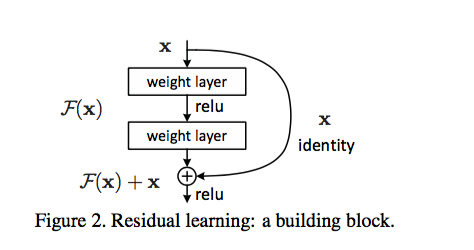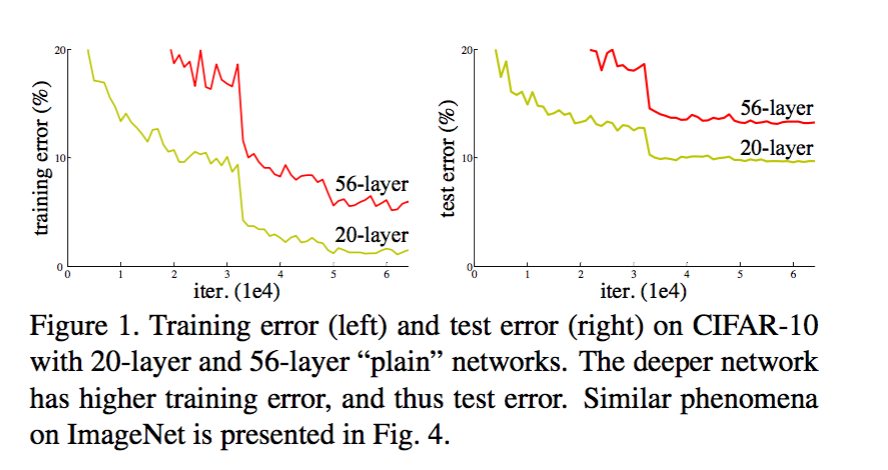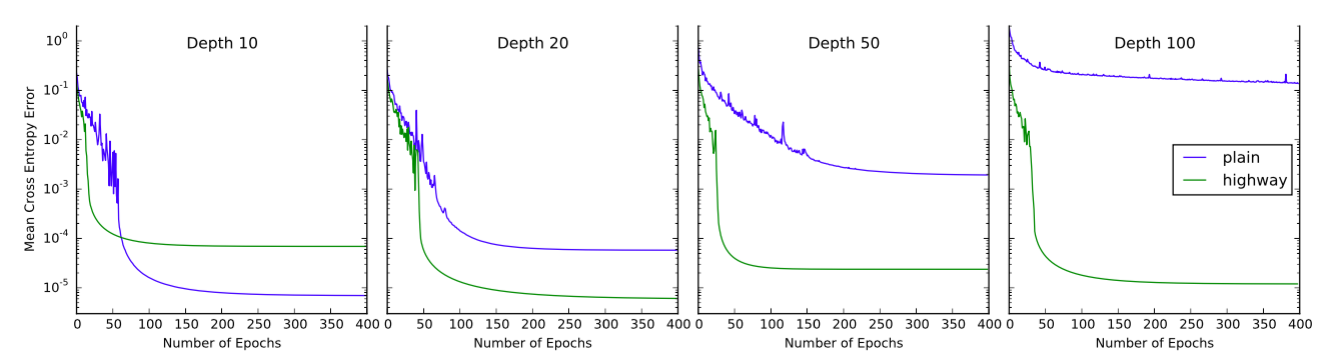# 深度残差网络和Highway网络

（来自论文《Deep Residual Learning for Image Recognition》）y=H(x,WH)T(x,WT)+xC(x,WC) $y=H(x,W_H)\bigodot T(x,W_T)+x\bigodot C(x,W_C)$
y $y$向量由两项组成。T$T$叫做transform gate ， C $C$叫做carry gate。C$C$ T $T$的激活函数都是sigmoid$sigmoid$函数。
T算出来的是一个向量 a1a2an $（a_1，a_2，……a_n）$,其中每个数字都是（0，1）之间的浮点数，代表y中由x变化后的内容所占的比例；
C算出来的也是一个向量 a1a2an $（a_1，a_2，……a_n）$,其中每个数字也都是（0，1）之间的浮点数，代表y中由x本身内容所占的比例；
（为了简便起见，有时候令 C=1T $C=1-T$ 1 $1$ 代表了维度和T$T$ 一样长的向量）从公式中我们需要注意的是，由于是点乘，当 C(x,WC) $C(x,W_C)$取了 1T(x,WT) $1-T(x,W_T)$之后那么 x,y,H(x,WH),T(x,WT) $x,y,H(x,W_H),T(x,W_T)$必须是同样的维度。如果我们想更改x的维度从A变成B的话，一种方法是采用zero-padding和下采样的方法，或者是引入一个维度为A*B的变换矩阵，使每次都乘上这个矩阵。A highway layer can smoothly vary its behavior between that of a plain layer and that of a layer which simply passes its inputs through.

02-23833508-302800
02-212803
09-033万+
04-062828
05-171万+
06-222万+
06-283937
04-24521
11-11525
04-093万+
12-152250
11-095814
04-07274
02-01654
07-251836
08-21391
02-211万+
09-283246